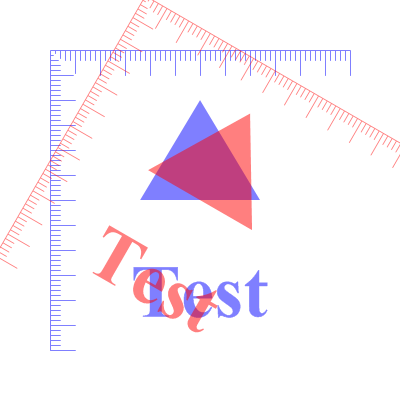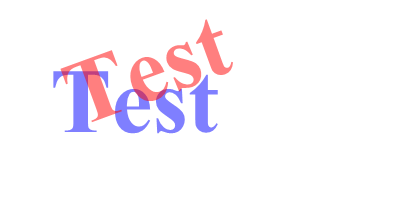RotateCoordinates()

Syntax

RotateCoordinates(x.d, y.d, Angle.d [, System])
Description
Rotate the vector drawing coordinate system around the given center point. The center point is expressed in terms of the current coordinate system.

Parameters

 x.d, y.d Specifies the center point for the rotation. Angle.d Specifies the rotation angle in degrees. A positive angle rotates the coordinate system clockwise. System (optional) Specifies the coordinate system to change. This can be one of the following values: ``` #PB_Coordinate_User : Change the coordinate system for points in the drawing path (default) #PB_Coordinate_Source: Change the coordinate system for the vector drawing source ```

Return value

None.

Remarks

See the vectordrawing overview for an introduction to the different coordinate systems.

The following image demonstrates the effect of rotated coordinates. The same figure is drawn twice, the original is in blue, and the version with rotated coordinates is in red.Example

```  If OpenWindow(0, 0, 0, 400, 200, "VectorDrawing", #PB_Window_SystemMenu | #PB_Window_ScreenCentered)
CanvasGadget(0, 0, 0, 400, 200)

If StartVectorDrawing(CanvasVectorOutput(0))
VectorFont(LoadFont(0, "Times New Roman", 60, #PB_Font_Bold))

VectorSourceColor(RGBA(0, 0, 255, 128))
MovePathCursor(50, 50)
DrawVectorText("Test")

RotateCoordinates(50, 50, -20) ; rotate by -20 degrees around the (50, 50) point

VectorSourceColor(RGBA(255, 0, 0, 128))
MovePathCursor(50, 50)
DrawVectorText("Test")

StopVectorDrawing()
EndIf

Repeat
Event = WaitWindowEvent()
Until Event = #PB_Event_CloseWindow
EndIf
```See Also

ResetCoordinates(), TranslateCoordinates(), ScaleCoordinates(), SkewCoordinates(), FlipCoordinatesX(), FlipCoordinatesY(), ConvertCoordinateX(), ConvertCoordinateY()

Supported OS

All

<- RestoreVectorState() - VectorDrawing Index - SaveVectorState() ->